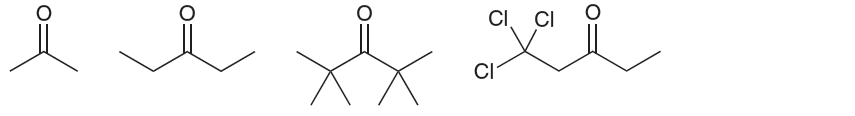Problem: In each group of compounds below, select the most acidic compound:

FREE Expert Solution
90% (386 ratings)
Problem Details

In each group of compounds below, select the most acidic compound:What scientific concept do you need to know in order to solve this problem?

Our tutors have indicated that to solve this problem you will need to apply the pKa concept. You can view video lessons to learn pKa Or if you need more pKa practice, you can also practice pKa practice problems .

What is the difficulty of this problem?

Our tutors rated the difficulty of In each group of compounds below, select the most acidic com... as medium difficulty.

How long does this problem take to solve?

Our expert Organic tutor, Jonathan took 2 minutes to solve this problem. You can follow their steps in the video explanation above.

What professor is this problem relevant for?

Based on our data, we think this problem is relevant for Professor Yuan's class at UCF.

What textbook is this problem found in?

Our data indicates that this problem or a close variation was asked in . You can also practice practice problems .